# Watering can

A watering can full of water weighs 11 kg, the weight of water is 10 kg greater than the weight of an empty watering can, how much is the weight of the watering can?

k =  0.5 kg

### Step-by-step explanation:

k+v=11
v = 10+k

k+v = 11
k-v = -10

k = 1/2 = 0.5
v = 21/2 = 10.5

Our linear equations calculator calculates it.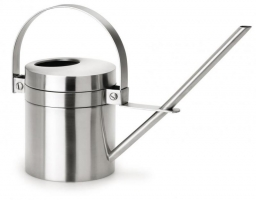Did you find an error or inaccuracy? Feel free to write us. Thank you!Tips to related online calculators
Do you have a linear equation or system of equations and looking for its solution? Or do you have a quadratic equation?

## Related math problems and questions:

• Can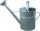Watering can full of water weighs 10 kg. Half-full can weighs 5.5 kg. How much weigh can?
• Water wessel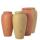The vessel containing water has a weight 19 kg. After pouring half the water its weigth 11 kg. What is the weight of the empty vessel?
• Bag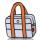Nelly found interesting point. An empty bag weighs 4 kg less than full. And empty bag is 5 times easier than full. How many kg weigh things in this bag?
• BucketThe bucket half filled with water weighs 5.55 kg, the full bucket weighs 9.85 kg. How much does the bucket weigh?
• Barrel 4Barrel of water weighs 63 kg. After off 75% water, the weight of the barrel with water is 21 kg. How many kg weigh empty barrel and how many kgs water in it?
• Water containerContainer with water weighs 1.48 kg. When we cast 75% of water container of water weight 0.73 kg. How heavy is an empty container?
• Empty canisterThe canister is filled with oil weight 17 kg. If filled only half weight 9 kg. What weight does my empty canister have?
• Container with water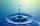The weight of a container with water is 2.48 kg. If the cast 75% water, the container with water has a weight of 0.98 kg. Determine the weight of the empty container. How much water was originally in the container?
• Barrel 2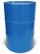Empty barrel weighs 7 kg. When it filled with water up to 45% height, weighs 60 kg. How heavy is barrel full of water?
• HomeworkMother and Kate together weigh 24.5 kg more than the father. Father weighs 7.6 kg more than mother. Father weight 44.8 kg more than Kate. Determine weights of father mother and Kate.
• Barrel with water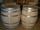The water barrel has a weight of 52 kg. When we pour a quarter of the water, the weight of the barrel is reduced to 40 kg. Determine the weight of the empty barrel.
• Two melons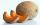There are 2 melons in the box. Together they weigh 11kg. The weight of green is 3 kg less than three times the weight of yellow. What is the weight of a green melon?
• Reservoir + waterThe reservoir filled with water weighs 12 kg and after pouring off three-quarters of the water weights 3 kg. Calculate the weight and volume of the reservoir.
• Barrel 3Barrel with water weights 118 kg. When we get off 75% of water, it weights 35 kg. How many kg has an empty barrel?
• Bob and Bobek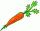Bobek has 2 cabbages and 5 carrots, Bob has 2 cabbages and 9 carrots. When Bobek stepped on the scale weight 5800 g and when Bob weight 8960 g. Actual Bob weight is 8 kg and Bobek 5 kg. How much weighs one carrot?
• Trio weight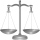Adelka, Barunka, and Cecilka are weight in pairs. Adelka with Barunka weighs 98 kg, Barunka with Cecilka 90 kg and Adelka with Cecilka 92 kg. How much does each of them weigh?
• CanisterGasoline is stored in a cuboid canister having dimensions 44.5 cm, 30 cm, 16 cm. What is the total weight of a full canister when one cubic meter of gasoline weighs 710 kg and the weight of empty canister is 1.5 kg?